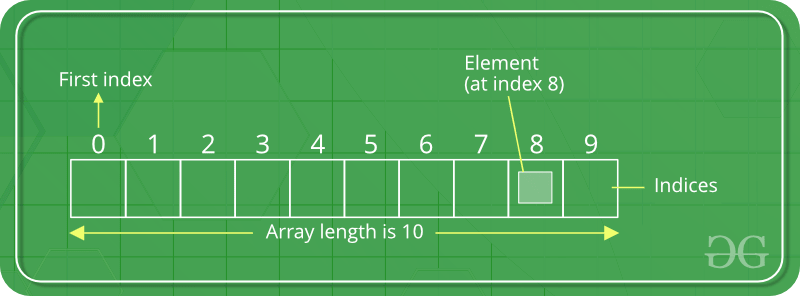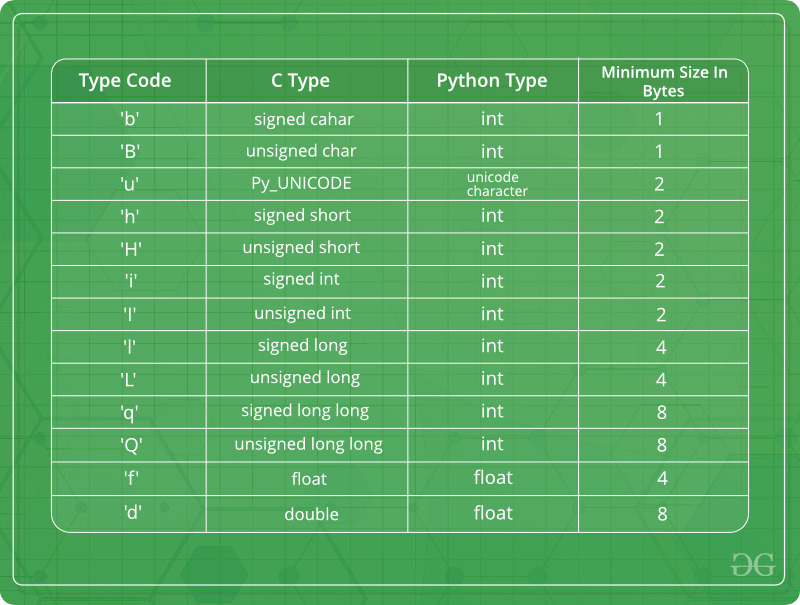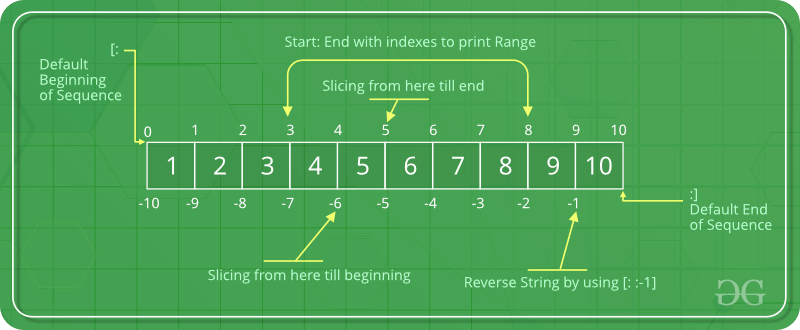# Python Arrays

An array is a collection of items stored at contiguous memory locations. The idea is to store multiple items of the same type together. This makes it easier to calculate the position of each element by simply adding an offset to a base value, i.e., the memory location of the first element of the array (generally denoted by the name of the array).

For simplicity, we can think of an array a fleet of stairs where on each step is placed a value (let’s say one of your friends). Here, you can identify the location of any of your friends by simply knowing the count of the step they are on. Array can be handled in Python by a module named `array`. They can be useful when we have to manipulate only a specific data type values. A user can treat lists as arrays. However, user cannot constraint the type of elements stored in a list. If you create arrays using the `array` module, all elements of the array must be of the same type.#### Creating a Array

Array in Python can be created by importing `array` module. `array(data_type, value_list)` is used to create an array with data type and value list specified in its arguments.

 `# Python program to demonstrate  ` `# Creation of Array  ` ` `  `# importing "array" for array creations ` `import` `array as arr ` ` `  `# creating an array with integer type ` `a ``=` `arr.array(``'i'``, [``1``, ``2``, ``3``]) ` ` `  `# printing original array ` `print` `(``"The new created array is : "``, end ``=``" "``) ` `for` `i ``in` `range` `(``0``, ``3``): ` `    ``print` `(a[i], end ``=``" "``) ` `print``() ` ` `  `# creating an array with float type ` `b ``=` `arr.array(``'d'``, [``2.5``, ``3.2``, ``3.3``]) ` ` `  `# printing original array ` `print` `(``"The new created array is : "``, end ``=``" "``) ` `for` `i ``in` `range` `(``0``, ``3``): ` `    ``print` `(b[i], end ``=``" "``) ` `    `

Output :

```The new created array is :  1 2 3
The new created array is :  2.5 3.2 3.3
```

Some of the data types are mentioned below which will help in creating an array of different data types.#### Adding Elements to a Array

Elements can be added to the Array by using built-in `insert()` function. Insert is used to insert one or more data elements into an array. Based on the requirement, a new element can be added at the beginning, end, or any given index of array. `append()` is also used to add the value mentioned in its arguments at the end of the array.

 `# Python program to demonstrate  ` `# Adding Elements to a Array ` ` `  `# importing "array" for array creations ` `import` `array as arr ` ` `  `# array with int type ` `a ``=` `arr.array(``'i'``, [``1``, ``2``, ``3``]) ` ` `  ` `  `print` `(``"Array before insertion : "``, end ``=``" "``) ` `for` `i ``in` `range` `(``0``, ``3``): ` `    ``print` `(a[i], end ``=``" "``) ` `print``() ` ` `  `# inserting array using  ` `# insert() function ` `a.insert(``1``, ``4``) ` ` `  `print` `(``"Array after insertion : "``, end ``=``" "``) ` `for` `i ``in` `(a): ` `    ``print` `(i, end ``=``" "``) ` `print``()  ` ` `  `# array with float type ` `b ``=` `arr.array(``'d'``, [``2.5``, ``3.2``, ``3.3``]) ` ` `  `print` `(``"Array before insertion : "``, end ``=``" "``) ` `for` `i ``in` `range` `(``0``, ``3``): ` `    ``print` `(b[i], end ``=``" "``) ` `print``() ` ` `  `# adding an element using append() ` `b.append(``4.4``) ` ` `  `print` `(``"Array after insertion : "``, end ``=``" "``) ` `for` `i ``in` `(b): ` `    ``print` `(i, end ``=``" "``) ` `print``()  `

Output :

```Array before insertion : 1 2 3
Array after insertion :  1 4 2 3
Array before insertion : 2.5 3.2 3.3
Array after insertion :  2.5 3.2 3.3 4.4
```

#### Accessing elements from the Array

In order to access the array items refer to the index number. Use the index operator [ ] to access an item in a array. The index must be an integer.

 `# Python program to demonstrate  ` `# accessing of element from list ` ` `  `# importing array module ` `import` `array as arr ` ` `  `# array with int type ` `a ``=` `arr.array(``'i'``, [``1``, ``2``, ``3``, ``4``, ``5``, ``6``]) ` ` `  `# accessing element of array ` `print``(``"Access element is: "``, a[``0``]) ` ` `  `# accessing element of array ` `print``(``"Access element is: "``, a[``3``]) ` ` `  `# array with float type ` `b ``=` `arr.array(``'d'``, [``2.5``, ``3.2``, ``3.3``]) ` ` `  `# accessing element of array ` `print``(``"Access element is: "``, b[``1``]) ` ` `  `# accessing element of array ` `print``(``"Access element is: "``, b[``2``]) `

Output :

```Access element is:  1
Access element is:  4
Access element is:  3.2
Access element is:  3.3
```

#### Removing Elements from the Array

Elements can be removed from the array by using built-in `remove()` function but an Error arises if element doesn’t exist in the set. `Remove()` method only removes one element at a time, to remove range of elements, iterator is used. `pop()` function can also be used to remove and return an element from the array, but by default it removes only the last element of the array, to remove element from a specific position of the array, index of the element is passed as an argument to the `pop()` method.

Note – Remove method in List will only remove the first occurrence of the searched element.

 `# Python program to demonstrate  ` `# Removal of elements in a Array ` `  `  `# importing "array" for array operations ` `import` `array ` `  `  `# initializing array with array values ` `# initializes array with signed integers ` `arr ``=` `array.array(``'i'``, [``1``, ``2``, ``3``, ``1``, ``5``])  ` ` `  `# printing original array ` `print` `(``"The new created array is : "``, end ``=``"") ` `for` `i ``in` `range` `(``0``, ``5``): ` `    ``print` `(arr[i], end ``=``" "``) ` ` `  `print` `(``"\r"``) ` ` `  `# using pop() to remove element at 2nd position ` `print` `(``"The popped element is : "``, end ``=``"") ` `print` `(arr.pop(``2``)) ` ` `  `# printing array after popping ` `print` `(``"The array after popping is : "``, end ``=``"") ` `for` `i ``in` `range` `(``0``, ``4``): ` `    ``print` `(arr[i], end ``=``" "``) ` ` `  `print``(``"\r"``) ` ` `  `# using remove() to remove 1st occurrence of 1 ` `arr.remove(``1``) ` ` `  `# printing array after removing ` `print` `(``"The array after removing is : "``, end ``=``"") ` `for` `i ``in` `range` `(``0``, ``3``): ` `    ``print` `(arr[i], end ``=``" "``) `

Output:

```The new created array is : 1 2 3 1 5
The popped element is : 3
The array after popping is : 1 2 1 5
The array after removing is : 2 1 5
```

#### Slicing of a Array

In Python array, there are multiple ways to print the whole array with all the elements, but to print a specific range of elements from the array, we use Slice operation. Slice operation is performed on array with the use of colon(:). To print elements from beginning to a range use [:Index], to print elements from end use [:-Index], to print elements from specific Index till the end use [Index:], to print elements within a range, use [Start Index:End Index] and to print whole List with the use of slicing operation, use [:]. Further, to print whole array in reverse order, use [::-1].`# Python program to demonstrate  ` `# silicing of elements in a Array ` ` `  `# importing array module ` `import` `array as arr ` ` `  `# creating a list  ` `l ``=` `[``1``, ``2``, ``3``, ``4``, ``5``, ``6``, ``7``, ``8``, ``9``, ``10``] ` ` `  `a ``=` `arr.array(``'i'``, l) ` `print``(``"Intial Array: "``) ` `for` `i ``in` `(a): ` `    ``print``(i, end ``=``" "``) ` ` `  `# Print elements of a range ` `# using Slice operation ` `Sliced_array ``=` `a[``3``:``8``] ` `print``(``"\nSlicing elements in a range 3-8: "``) ` `print``(Sliced_array) ` ` `  `# Print elements from a  ` `# pre-defined point to end ` `Sliced_array ``=` `a[``5``:] ` `print``(``"\nElements sliced from 5th "` `      ``"element till the end: "``) ` `print``(Sliced_array) ` ` `  `# Printing elements from ` `# beginning till end ` `Sliced_array ``=` `a[:] ` `print``(``"\nPrinting all elements using slice operation: "``) ` `print``(Sliced_array) `

Output :

```Intial Array:
1 2 3 4 5 6 7 8 9 10
Slicing elements in a range 3-8:
array('i', [4, 5, 6, 7, 8])

Elements sliced from 5th element till the end:
array('i', [6, 7, 8, 9, 10])

Printing all elements using slice operation:
array('i', [1, 2, 3, 4, 5, 6, 7, 8, 9, 10])
```

#### Searching element in a Array

In order to search an element in the array we use a python in-built `index()` method. This function returns the index of the first occurrence of value mentioned in arguments.

 `# Python code to demonstrate  ` `# searching an element in array ` ` `  `  `  `# importing array module ` `import` `array ` `  `  `# initializing array with array values ` `# initializes array with signed integers ` `arr ``=` `array.array(``'i'``, [``1``, ``2``, ``3``, ``1``, ``2``, ``5``])  ` ` `  `# printing original array ` `print` `(``"The new created array is : "``, end ``=``"") ` `for` `i ``in` `range` `(``0``, ``6``): ` `    ``print` `(arr[i], end ``=``" "``) ` ` `  `print` `(``"\r"``) ` ` `  `# using index() to print index of 1st occurrenece of 2 ` `print` `(``"The index of 1st occurrence of 2 is : "``, end ``=``"") ` `print` `(arr.index(``2``)) ` ` `  `# using index() to print index of 1st occurrenece of 1 ` `print` `(``"The index of 1st occurrence of 1 is : "``, end ``=``"") ` `print` `(arr.index(``1``)) `

Output:

```The new created array is : 1 2 3 1 2 5
The index of 1st occurrence of 2 is : 1
The index of 1st occurrence of 1 is : 0
```

#### Updating Elements in a Array

In order to update an element in the array we simply reassign a new value to the desired index we want to update.

 `# Python code to demonstrate  ` `# how to update an element in array ` ` `  `# importing array module ` `import` `array ` ` `  `# initializing array with array values ` `# initializes array with signed integers ` `arr ``=` `array.array(``'i'``, [``1``, ``2``, ``3``, ``1``, ``2``, ``5``])  ` ` `  `# printing original array ` `print` `(``"Array before updation : "``, end ``=``"") ` `for` `i ``in` `range` `(``0``, ``6``): ` `    ``print` `(arr[i], end ``=``" "``) ` ` `  `print` `(``"\r"``) ` ` `  `# updating a element in a array ` `arr[``2``] ``=` `6` `print``(``"Array after updation : "``, end ``=``"") ` `for` `i ``in` `range` `(``0``, ``6``): ` `    ``print` `(arr[i], end ``=``" "``) ` `print``() ` ` `  `# updating a element in a array ` `arr[``4``] ``=` `8` `print``(``"Array after updation : "``, end ``=``"") ` `for` `i ``in` `range` `(``0``, ``6``): ` `    ``print` `(arr[i], end ``=``" "``) `

Output:

```Array before updation : 1 2 3 1 2 5
Array after updation : 1 2 6 1 2 5
Array after updation : 1 2 6 1 8 5
```

Attention geek! Strengthen your foundations with the Python Programming Foundation Course and learn the basics.

To begin with, your interview preparations Enhance your Data Structures concepts with the Python DS Course.

My Personal Notes arrow_drop_upIf you like GeeksforGeeks and would like to contribute, you can also write an article using contribute.geeksforgeeks.org or mail your article to contribute@geeksforgeeks.org. See your article appearing on the GeeksforGeeks main page and help other Geeks.

Please Improve this article if you find anything incorrect by clicking on the "Improve Article" button below.

Article Tags :

18

Please write to us at contribute@geeksforgeeks.org to report any issue with the above content.